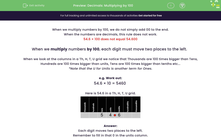# Practise Multiplying Decimals by 100

In this worksheet, students multiply numbers by 100 by shifting digits two places to the left in a grid.Key stage:  KS 2

Curriculum topic:   Number: Multiplication and Division

Curriculum subtopic:   Multiply/Divide Using Decimals

Popular topics:   Decimals worksheets, Place Value worksheets

Difficulty level:#### Worksheet Overview

When we multiply numbers by 100, we do not simply add 00 to the end.

When the numbers are decimals, this rule does not work.

54.6 × 100 does not equal 54.600

When we multiply numbers by 100, each digit must move two places to the left.

When we look at the columns in a Th, H, T, O grid we notice that thousands are 100 times bigger than tens, hundreds are 100 times bigger than ones, tens are 100 times bigger than tenths etc...

e.g. Work out:

54.6 × 100 = 5460

Here is 54.6 in a decimal place value grid.5 4 . 6

Each digit moves two places to the left.

Remember to fill in that 0 in the ones column.

So  54.6 × 100 = 5460

### What is EdPlace?

We're your National Curriculum aligned online education content provider helping each child succeed in English, maths and science from year 1 to GCSE. With an EdPlace account you’ll be able to track and measure progress, helping each child achieve their best. We build confidence and attainment by personalising each child’s learning at a level that suits them.

Get started